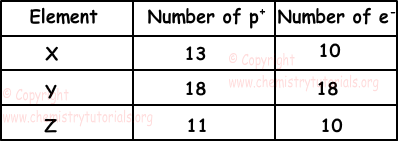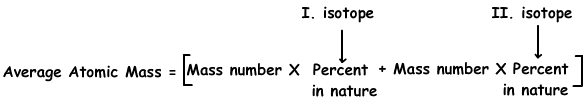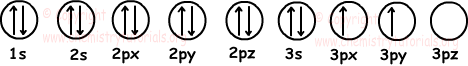EN | ES | DE | NL | RU

## Atomic Structure Exam2 and Problem Solutions

Atomic Structure Exam2 and  Problem Solutions

1. Which ones of the following relations about 714X-3 ion are true?

I. p+=n0

II. e->p+

III. Mass Number =p+ + e-

Solution:

Atomic number=number of protons =7

p+=7

Mass number=p+ + n0

14=7+n0

n0=7

n0=p+=7 I is true

We find charge of ion as;

charge of ion=p+ - e-

-3=7-e-

e-=10 (e->p+) II is true

Mass number is 14 on the contrary, sum of p+ and e- is 17

III is false.

2. Positively charged ions are called cation and negatively charged ions are called anion. Table given below shows number of electrons and protons of given elements.Which ones of the elements given above are cation?

Solution:

In a neutral atom number of protons is equal to number of electrons.

Number of protons of X is larger than number of electrons, so X is cation.

Number of protons of Y is equal to number of electrons, so Y is neutral.

Number of protons of Z is larger than number of electrons, so Z is cation.

3. If number of electrons of X+3, Y-3 and Z are equal, which ones of the following statements are true for them.

I. X has largest nuclear charge

II. Volume of Y-3 ion is larger than others

III. Attraction of one electron;

X+3> Z > Y-3

Solution:

Let me show number of electrons with "a". Charge of ion can e found with following equation;

charge=p+-e-

For X+3 ion;

+3=p+-a then, p+=a+3 and a+3X+3

For Y-3 ion;

-3 = p+-a then, p+=a-3 and a-3Y-3

For Z atom;

p+=e- then p+=a and aZ0

charge of nucleus is equal to number of protons. So, X has largest nuclear charge. I is true.

Since they have equal number of electrons, attraction of one electron is directly proportional to number of protons. If number of protons increases then, attraction of electrons also increases. Thus, relation becomes;

X+3 > Z > Y-3 III is true

Volume is inversely proportional to attraction of one electron.

X+3 < Z < Y-3 II is true

4. Cu has two isotopes; 63Cu in 70 % and 65Cl in 30%. Find average atomic mass of Cu atom.

Solution:

We use following formula to fşnd average atomic mass of Cu;Average atomic mass of Cu=63.(70/100) + 65.(30/100)

Average atomic mass of Cu=63,6 g

5. Find Atomic number of element that has electron configuration lasting with 6p2.

Solution:

To find atomic number of element, we should write all orbitals until 6p2 and sum number of electrons.

1s22s22p63s23p64s23d104p65s24d105p66s24f145d106p2

Sum of number of electrons is 82. So it also has 82 protons and atomic number is 82.

6. Find number of filled and half filled orbitals of 14Si element.

Solution:

We first write electron configuration of 14Si and then show electrons in orbitals.

14Si: 1s22s22p63s23p2

Orbitals;As you can see from the given diagram, 14Si has 6 filled and 2 half filled orbitals.

The Original Author: